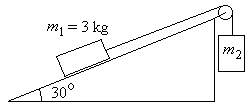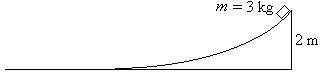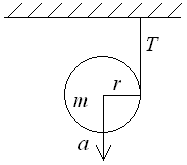First Name: ___________________ Last Name: ____________________ Section: _________

October 23, 1998 Physics 207

Exam 2

Print your name and section clearly on all five pages. (If you do not know your section number, write your TAs name.) Show all work in the space immediately below each problem. Your final answer must be placed in the box provided. Problems will be graded on reasoning and intermediate steps as well as on the final answer. Be sure to include units wherever necessary, and the direction of vectors. Each problem is worth 25 points. In doing the problems, try to be neat. Check your answers to see that they have the correct dimensions (units) and are the right order of magnitudes. You are allowed one 8½ x 11" sheet of notes and no other references. The exam lasts exactly 50 minutes.

(Do not write below)

SCORE:

Problem 1: __________

Problem 2: __________

Problem 3: __________

Problem 4: __________

TOTAL: ___________

First Name: ___________________ Last Name: ____________________ Section: _________

1. A 3-kg mass rests on a plane inclined at an angle of 30° as shown and has a coefficient of static friction µS = 0.4.a. What is the largest mass m2 that can be attached to the string passing over the pulley without causing the mass m1 to move ? (10 pts.)

b. If the mass m2 is changed to 5 kg and the block accelerates up the plane with a = 3.3 m/s2, what is the coefficient of kinetic friction mk? (15 pts.)

First Name: ___________________ Last Name: ____________________ Section: _________

2. A block with mass m = 3 kg is released from rest 2 m above the ground and slides down a track with friction as shown.a. If the block reaches the ground with a speed of 4 m/s, how much work is done on the block by friction? (9 pts.)

b. If the block continues to slide along the ground with a constant deceleration, coming to rest after traveling 1.5 m along the ground, what is the coefficient of friction between the block and the ground? (8 pts.)

c. What is the average power produced by the friction force while the block is sliding horizontally along the ground? (8 pts.)

First Name: ___________________ Last Name: ____________________ Section: _________

3. A 2000-kg automobile moving 30 m/s collides head-on with a 3000-kg truck initially at rest, and the automobile comes to rest just after the collision.

1. What is the speed of the truck just after the collision? (6 pts.)

2.

3. What fraction of the mechanical energy is lost in the collision? (7 pts.)

c. What is the impulse on the truck? (6 pts.)

d. If the collision lasts 0.5 s, what is the average force on the truck? (6 pts.)

First Name: ___________________ Last Name: ____________________ Section: _________

4. A yo-yo consists of a uniform cylinder of radius r = 10 cm and mass m = 100 g suspended by a string from above as shown.a. What is the downward acceleration a of the center of mass of the yo-yo? (9 pts.)

b. What is the tension T in the string? (8 pts.)

c. Through how many radians does the yo-yo rotate in the first second after being released from rest? (8 pts.)